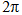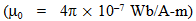# Two concentric coils each of radius equal to  cm are placed at right angles to each other. 3 ampere and 4 ampere are the currents flowi...

Two concentric coils each of radius equal tocm are placed at right angles to each other. 3 ampere and 4 ampere are the currents flowing in each coil respectively. The magnetic induction in weber/m2 at the center of the coils will beAnonymous User Physics Magnetic Effect of Current 16 Apr, 2020 53 views